Chapter 2, Problem 1RCC

Chapter
Section
Textbook Problem

Explain what each of the following means and illustrate with sketch.(a) lim x → a f ( x ) = L (b) lim x → a + f ( x ) = L (c) lim x → a − f ( x ) = L (d) lim x → a f ( x ) = ∞ (e) lim x → ∞ f ( x ) = L

(a)

To determine

To explain: The meaning of limxaf(x)=L.

Explanation

Result used:

Definition of limit:

Let f(x) be a function is defined when x approaches to p then limxpf(x)=L, if for every number ε>0 there is some δ>0  such that |f(x)L|<ε whenever 0<|xp|<δ.

Graph: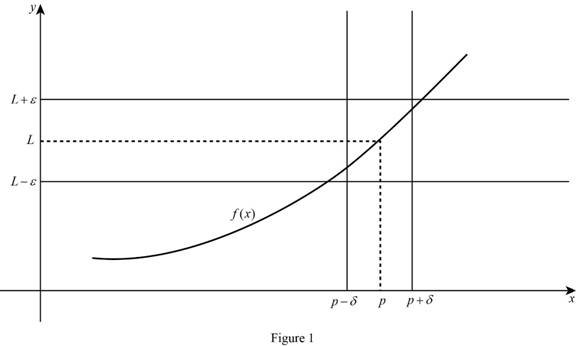Calculation:

The limit of the function limxaf(x)=L means the limit of f(x) equal to L when x approaches to a, if x is closer and closer to a from the both sides then the value of f(x) also closer and closer to L.

In the limit definition xa this means finding the limit of f(x) when x approaches to a, there no need to consider x=a.

There are three cases for define limxaf(x)=L.

Case (1):

The limit of the function limxaf(x)=L, if x approaches to a then the value of f(x) are closer to L and f(a) is L.

Graph: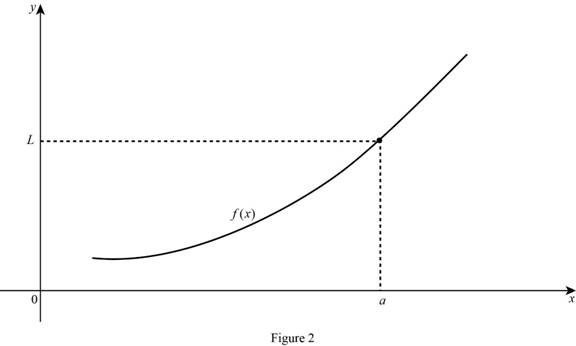Case (2):

The limit of the function limxaf(x)=L, if x approaches to a then the value of f(x) are closer to L and f(a) is undefined.

Case (3):

The limit of the function limxaf(x)=L, if x approaches to a then the value of f(x) are closer to other than L.

Graph: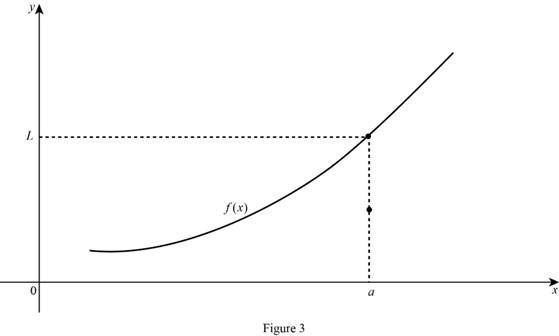(b)

To determine

To explain: The meaning of limxa+f(x)=L.

Explanation

Result used:

Definition of limit:

Let f(x) be a function is defined when x approaches to p then limxpf(x)=L, if for every number ε>0 there is some δ>0  such that |f(x)L|<ε whenever 0<|xp|<δ.

Calculation:

limxa+f(x)=L means the limit of f(x) equal to L when x approaches to a from the right, if x is closer and closer to a from the right and remains greater than a then the value of f(x) also closer and closer to L.

Graph: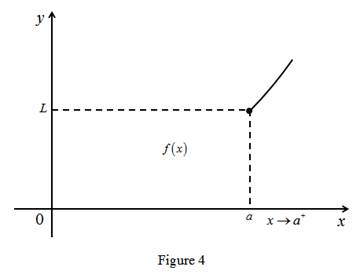(c)

To determine

To explain: The meaning of limxaf(x)=L.

Explanation

Result used:

Definition of limit:

Let f(x) be a function is defined when x approaches to p then limxpf(x)=L, if for every number ε>0 there is some δ>0  such that |f(x)L|<ε whenever 0<|xp|<δ.

Calculation:

The limit of the function limxaf(x)=L means the limit of f(x) equal to L when x approaches to a from the left, if x is closer and closer to a from the left and remains less than a then the value of f(x) also closer and closer to L.

Graph: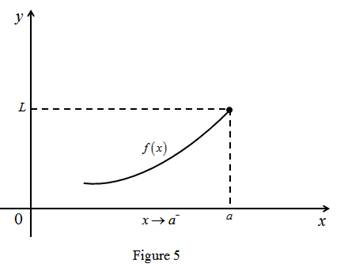(d)

To determine

To explain: The meaning of limxaf(x)=.

Explanation

Result used:

Definition of limit:

Let f(x) be a function is defined when x approaches to p then limxpf(x)=L, if for every number ε>0 there is some δ>0  such that |f(x)L|<ε whenever 0<|xp|<δ.

Calculation:

The limit of the function limxaf(x)= means the limit of f(x) is larger value when x approaches to a from the both sides. That is any M>0, f(x)>M for some x-value is sufficiently close to a.

Graph: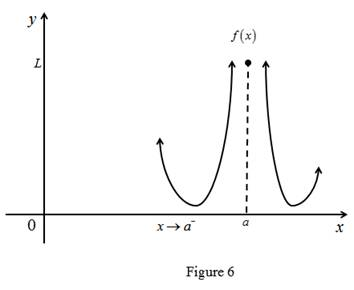(e)

To determine

To explain: The meaning of limxf(x)=L.

Explanation

Result used:

Definition of limit:

Let f(x) be a function is defined when x approaches to p then limxpf(x)=L, if for every number ε>0 there is some δ>0  such that |f(x)L|<ε whenever 0<|xp|<δ.

Calculation:

The limit of the function limxf(x)=L means the limit of f(x) is L when x approaches to larger value the graph get closer and closer to the line y=L.

Graph: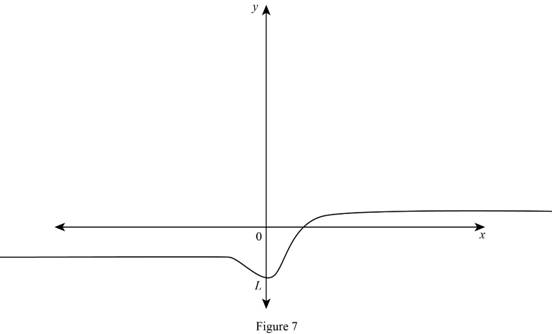The Solution to Your Study Problems

Bartleby provides explanations to thousands of textbook problems written by our experts, many with advanced degrees!

Get Started

Solve each inequality for x. 56. (a) 1 e3x1 2 (b) 1 2 ln x 3

Single Variable Calculus: Early Transcendentals, Volume I

Construct an equilateral triangle ABC with side AB.

Elementary Geometry For College Students, 7e

Find all the answers to your study problems with bartleby.
Textbook solutions plus Q&A. Get As ASAP arrow_forward